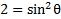# Understanding the Pythagorean Identities

There are three main Pythagorean identities: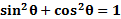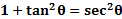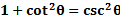These are derived from the Pythagorean Theorem and the unit circle. And they are very useful when for manipulating and solving equations.

Before you can use these to solve equations, you must understand how to manipulate them.

Examples:

1) Simplify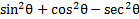using the Pythagorean identities

Since we know that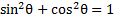we can replace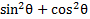with 1: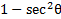This is good, but we can go even further. If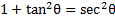then we can rearrange this identity by moving the 1 to the other side: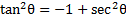and then multiplying both sides by -1: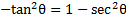. Thus, our expression actually equals: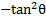Being able to manipulate expressions will be helpful when solving more complex equations.

2) Rewrite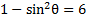to contain a cos function instead of a sin function.

We can replace 1 with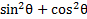: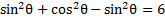Now we can add like terms.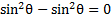. Thus our equation is now: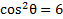3) Simplify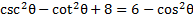and rewrite it to contain only a sin function.
First, since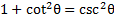, let's replace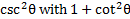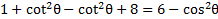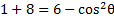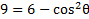We know that, but there is no 1 to replace. However, if we subtract 5 from both sides then there will be.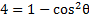Now we can substitutefor the 1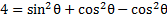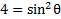Practice: Use the Pythagorean Identities to rewrite the following expressions as instructed:

1) Simplify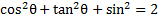2) Simplify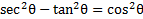3) Rewrite this expression to include sin instead of cos: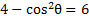4) Rewrite this expression to include cot instead of csc: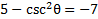5) Simplify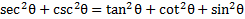1)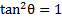2)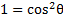3)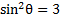4)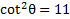5)